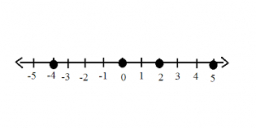# Consecutive 66034

There are five consecutive natural numbers. If the middle number is subtracted from the sum of the other four, I get 21. Specify these numbers.

a =  5
b =  6
c =  7
d =  8
e =  9

### Step-by-step explanation:Did you find an error or inaccuracy? Feel free to write us. Thank you!

Tips for related online calculators
Do you have a linear equation or system of equations and looking for its solution? Or do you have a quadratic equation?